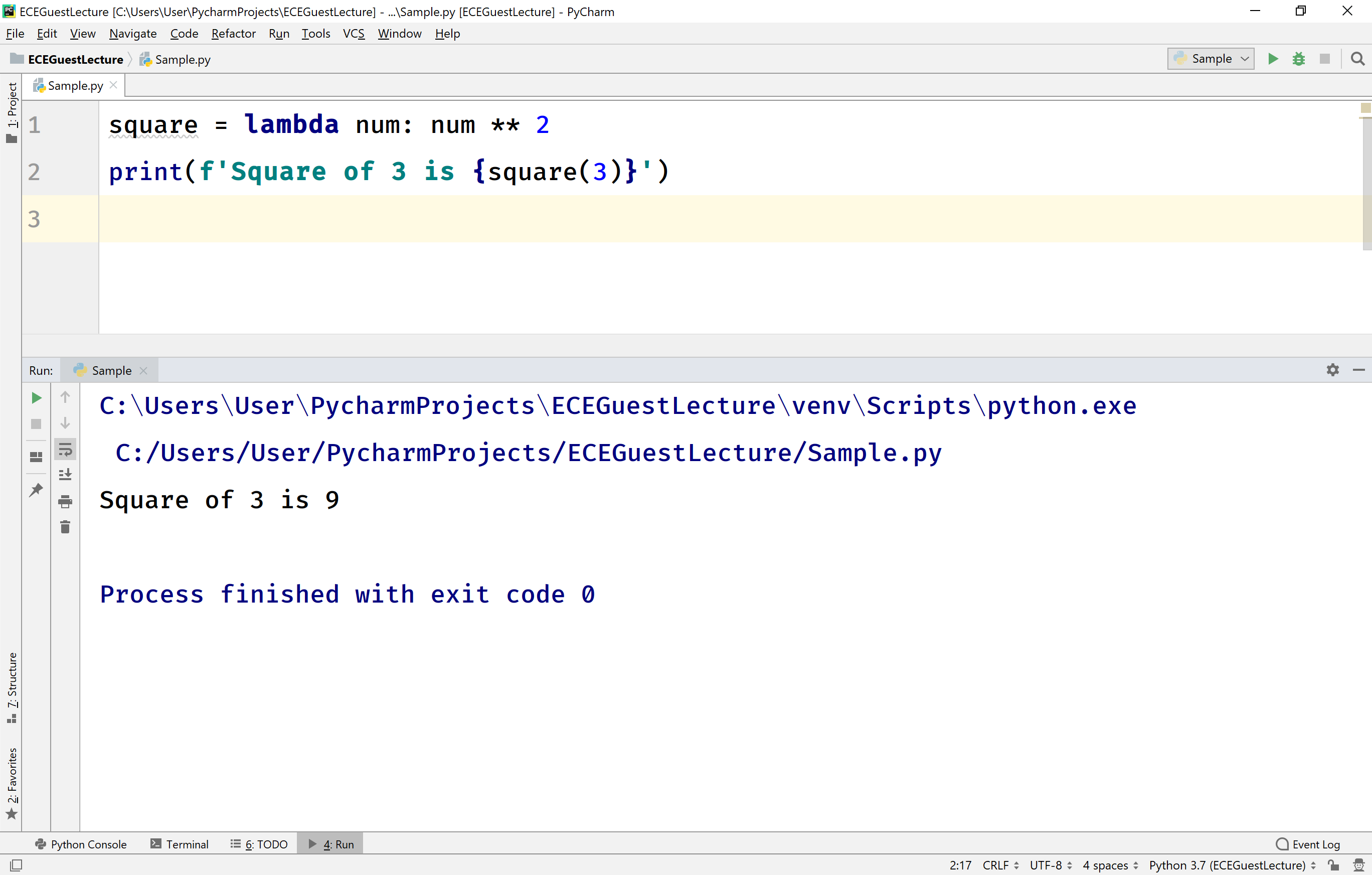The perfect place for easy learning...

×

# Python Lambda Expressions

In Python, the lambda expression is an anonymous function. In other words, the lambda expression is a function that is defined without a name. Some times the lambda expression is also said to be lambda function. The general syntax to define lambda function is as follows.

### Syntax

lambda arguments: expression

The lambda function can take any number of arguments but contains only one expression and the result of that expression is returned as a return value.

Points to be Remembered!
• The keyword lambda is used to create lambda expressions.
• The lambda expression can have any number of arguments
• The lambda expression must contain only one expression which is evaluated and returned.
• The lambda expression is called using the name of the variable to which the lambda expression has assigned.
• The lambda expression does not use the keyword return, it automatically returns the result of the expression.
• We can use the lambda expression anywhere a function is expected. Always we don't have to assign it to a variable.

Consider the following example code.

# Python code to illustrate lambda function.

``````square = lambda num: num ** 2
print(f'Square of 3 is {square(3)}')```
```

In the above example code, lambda is the keyword used to create lambda expression. The num is an argument to the lambda function, and num ** 2 is the expression in the lambda function. Every lambda function is called using its destination variable name. Here we have called it using the statement "square(3)".

When we run the above example code, it produces the following output.Consider the following example code.

# Python code to illustrate lambda function.

``````total = lambda n1, n2, n3: n1 + n2 + n3
print(f'total = {total(10, 20, 30)}')```
```

In the above example code, the lambda expression used with three arguments n1, n2, and n3.

When we run the above example code, it produces the following output.The lambda expressions are used with built-in functions like map, filter, and reduce. When the lambda expression is used with these built-in functions, it doesn't have to be assigned to a variable.